# IB DP Chemistry: SL复习笔记6.1.3 Measuring Rates

### Measuring Rates

• To measure the rate of a reaction, we need to be able to measure either how quickly the reactants are used up or how quickly the products are formed
• The method used for measuring depends on the substances involved
• There are a number of ways to measure a reaction rate in the lab; they all depend on some property that changes during the course of the reaction
• That property is taken to be proportional to the concentration of the reactant or product, e.g., colour, mass, volume
• Some reaction rates can be measured as the reaction proceeds (this generates more data);
• faster reactions can be easier to measure when the reaction is over, by averaging a collected measurement over the course of the reaction
• Three commonly used techniques are:
• mass loss
• gas production
• colorimetry

#### Changes in colour

• A colorimeter measures the amount of light that passes through a solution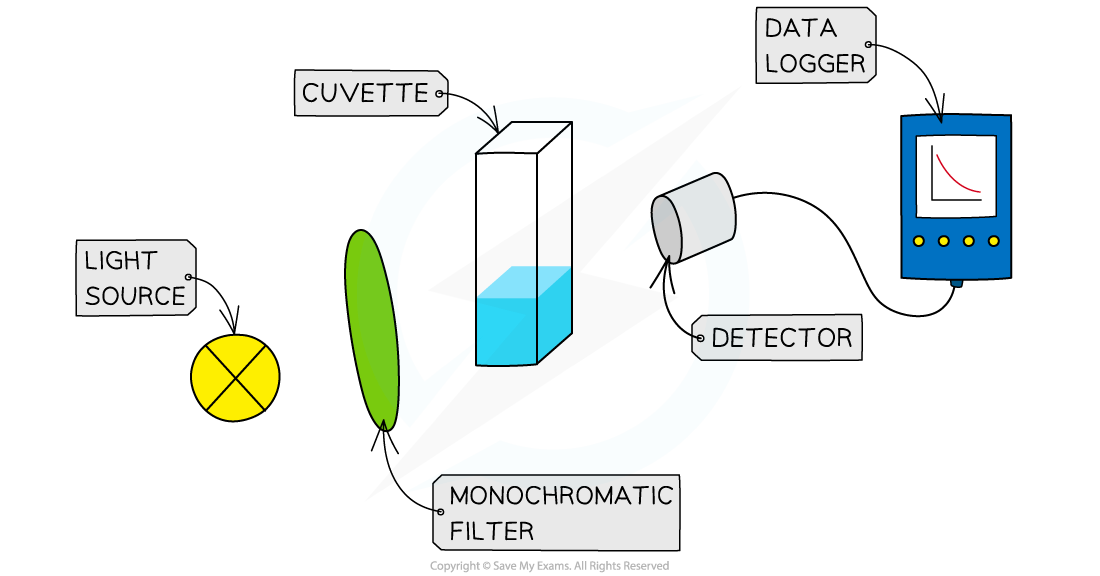Colorimetry Set Up

• If a solution changes colour during a reaction this can be used to measure the rate
• The intensity of light reaching the detector is measured every few seconds and the data is plotted to show how the concentration of the reactants or products changes with time
• The light intensity is related to the concentration, so the graph represents a graph of concentration of products or reactants against time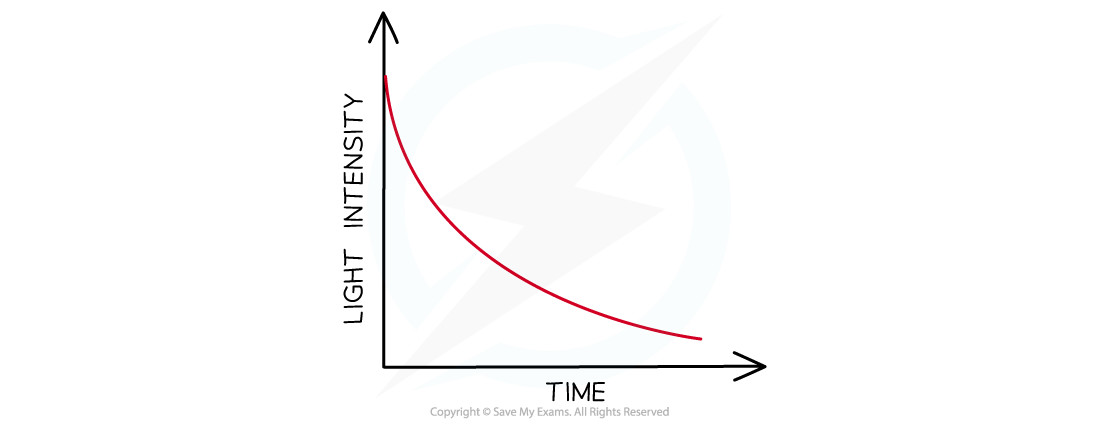Sketch graph of colour intensity against time (the coloured species is a reactant in this case)

• Note that colorimetry cannot be used to monitor the formation of coloured precipitates as the light will be scattered or blocked by the precipitate

#### Changes in mass

• When a gas is produced in a reaction it usually escapes from the reaction vessel, so the mass decreases
• This can be used to measure the rate of reaction
• For example, the reaction of calcium carbonate with hydrochloric acid produces CO2
• The mass is measured every few seconds and change in mass over time is plotted as the CO2 escapes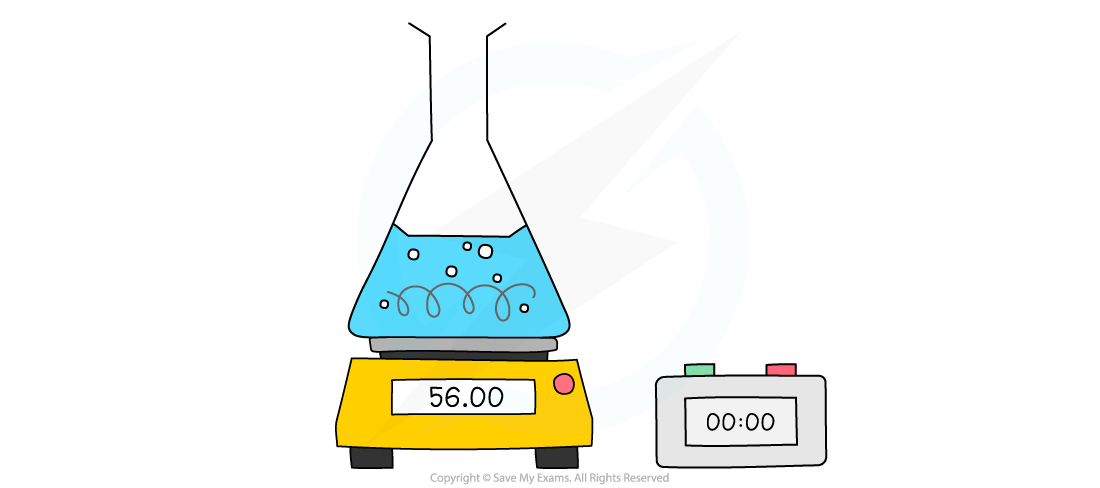Measuring changes in mass using a balance

• The mass loss provides a measure of the amount of reactant, so the graph is the same as a graph of amount of reactant against time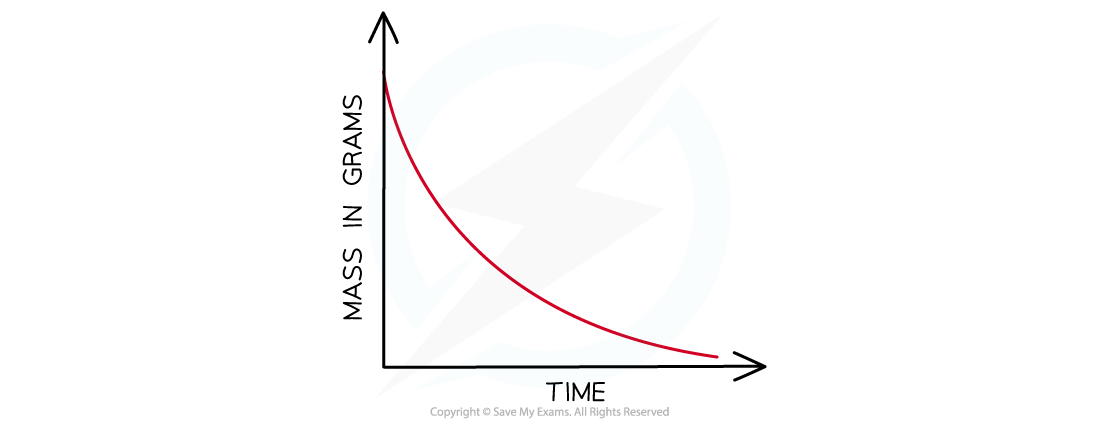Mass loss of a product against time

• However, one limitation of this method is the gas must be sufficiently dense or the change in mass is too small to measure on a 2 or 3 dp balance
• So carbon dioxide would be suitable (Mr = 44) but hydrogen would not (Mr = 2)

#### Volumes of gases

• When a gas is produced in a reaction, it can be trapped and its volume measured over time
• This can be used to measure the rate of reaction.
• For example, the reaction of magnesium with hydrochloric acid produces hydrogen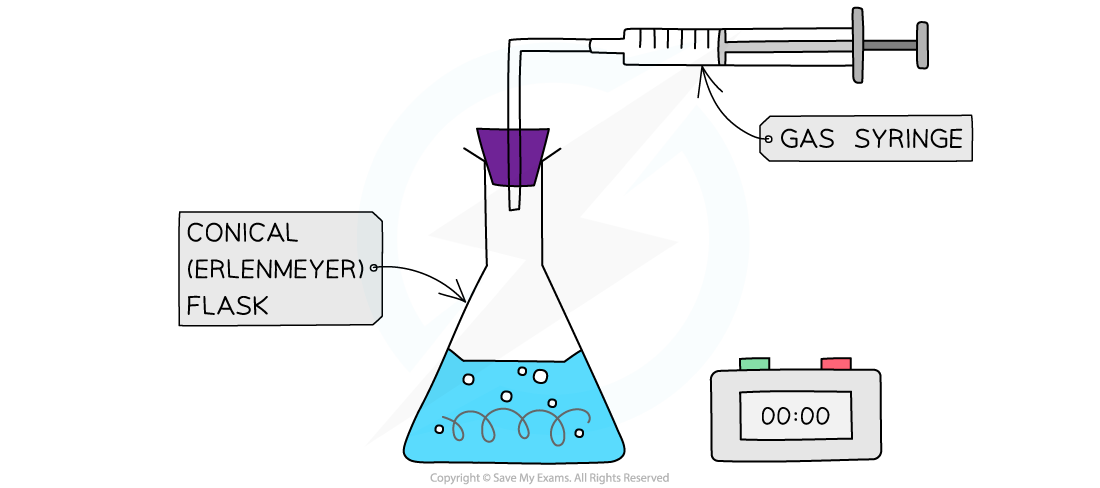Collecting gases experimental set up

• An alternative gas collection set up involves collecting a gas through water using an inverted measuring cylinder ( as long as the gas is not water soluble)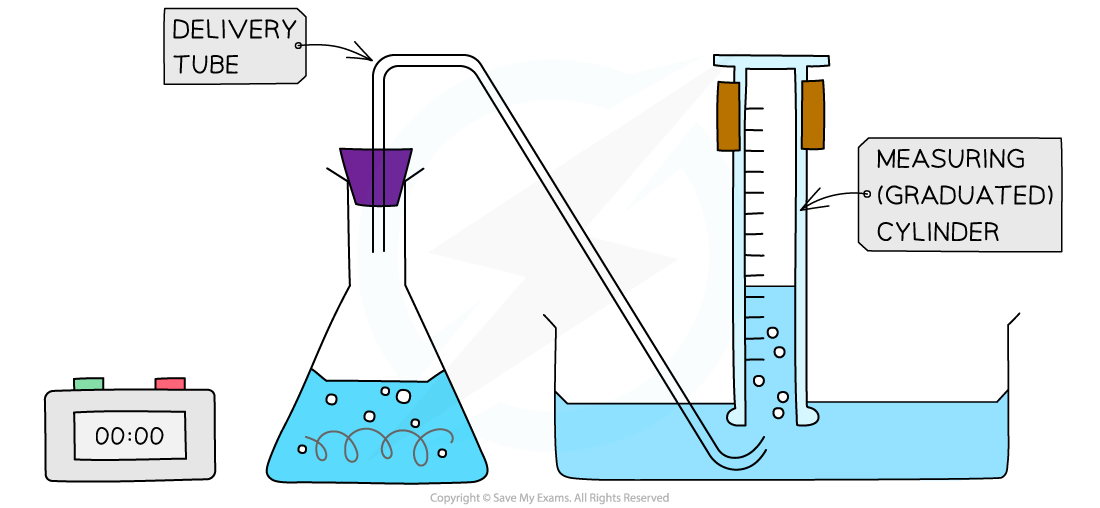Alternative gas collection set up

• The volume can be measured every few seconds and plotted to show how the volume of gas varies with time
• The volume provides a measure of the amount of product, so the graph is a graph of amount of product against time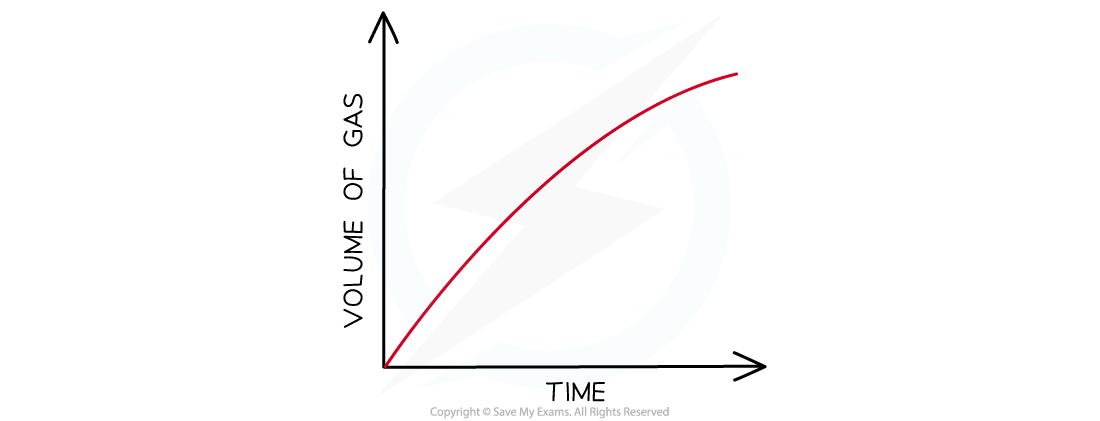Graph of gas volume evolved against time

#### Measuring concentration changes

• Measuring concentration changes during a reaction is not easy; the act of taking a sample and analysing it by titration can affect the rate of reaction (unless the reaction is deliberately stopped- this is called quenching).
• Often it is more convenient to ‘stop the clock’ when a specific (visible) point in the reaction is reached
• For example when a piece of magnesium dissolves completely in hydrochloric acid
• Another common rate experiment is the reaction between sodium thiosulfate and hydrochloric acid which slowly produces a yellow precipitate of sulfur that obscures a cross when viewed through the solution:

Na2S2O(aq) + 2HCl (aq)   →  2NaCl aq) + SO2 (g) + H2O (l) + S(s)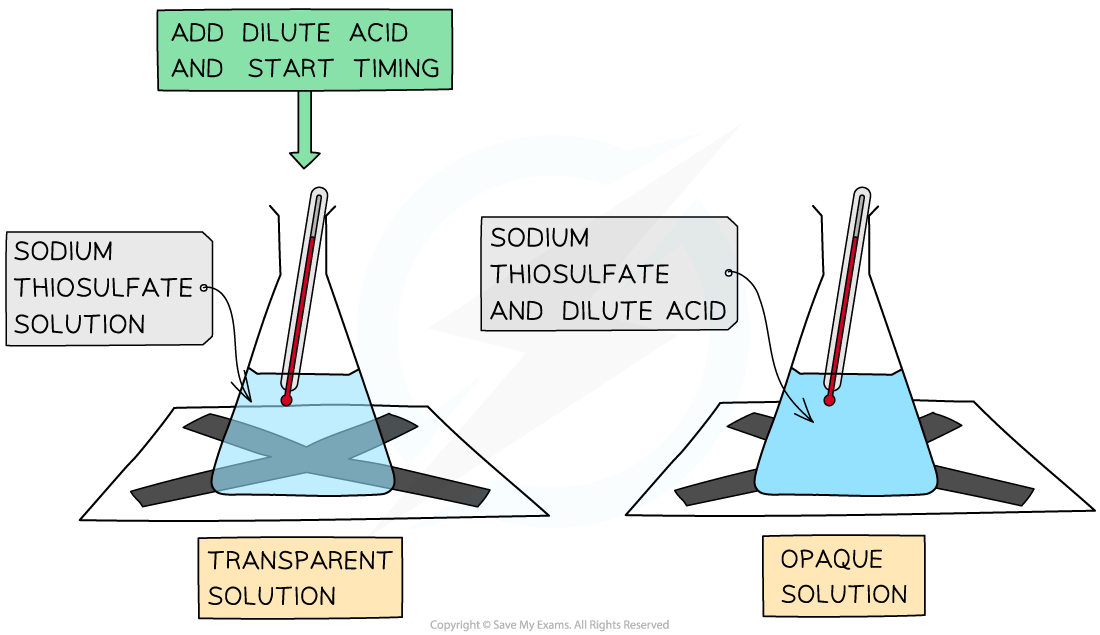The disappearing cross experiment

• The main limitation here is that often it only generates one piece of data for analysis

#### Exam Tip

You should be familiar with the interpretation of graphs of changes in concentration, volume or mass against time and be able to calculate a rate from a tangent to the graph